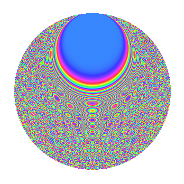# Properties

 Label 756.2.bsLevel 756 Weight 2 Character orbit bs Rep. character $$\chi_{756}(11,\cdot)$$ Character field $$\Q(\zeta_{18})$$ Dimension 840 Newform subspaces 1 Sturm bound 288 Trace bound 0

# Related objects

## Defining parameters

 Level: $$N$$ = $$756 = 2^{2} \cdot 3^{3} \cdot 7$$ Weight: $$k$$ = $$2$$ Character orbit: $$[\chi]$$ = 756.bs (of order $$18$$ and degree $$6$$) Character conductor: $$\operatorname{cond}(\chi)$$ = $$756$$ Character field: $$\Q(\zeta_{18})$$ Newform subspaces: $$1$$ Sturm bound: $$288$$ Trace bound: $$0$$

## Dimensions

The following table gives the dimensions of various subspaces of $$M_{2}(756, [\chi])$$.

Total New Old
Modular forms 888 888 0
Cusp forms 840 840 0
Eisenstein series 48 48 0

## Trace form

 $$840q - 3q^{2} - 3q^{4} - 6q^{5} - 12q^{6} - 18q^{8} - 6q^{9} + O(q^{10})$$ $$840q - 3q^{2} - 3q^{4} - 6q^{5} - 12q^{6} - 18q^{8} - 6q^{9} - 6q^{10} - 3q^{12} - 24q^{13} - 21q^{14} - 3q^{16} - 3q^{18} - 12q^{20} - 12q^{21} - 12q^{22} - 15q^{24} - 6q^{25} - 12q^{28} - 12q^{29} + 33q^{30} + 27q^{32} - 6q^{33} - 6q^{34} - 42q^{36} + 6q^{37} - 9q^{38} + 12q^{40} - 24q^{41} - 21q^{42} - 9q^{44} - 30q^{45} + 3q^{46} - 78q^{48} - 12q^{49} - 27q^{50} - 3q^{52} + 39q^{54} - 27q^{56} - 6q^{57} - 3q^{58} + 27q^{60} - 6q^{61} - 117q^{62} - 6q^{64} + 18q^{65} - 3q^{66} + 60q^{68} - 48q^{69} + 24q^{70} - 63q^{72} + 6q^{73} - 75q^{74} + 12q^{77} + 6q^{78} + 18q^{81} - 6q^{82} + 132q^{84} + 6q^{85} - 81q^{86} + 45q^{88} - 39q^{90} + 48q^{92} - 6q^{93} - 3q^{94} - 111q^{96} - 24q^{97} - 9q^{98} + O(q^{100})$$

## Decomposition of $$S_{2}^{\mathrm{new}}(756, [\chi])$$ into newform subspaces

Label Dim. $$A$$ Field CM Traces $q$-expansion
$$a_2$$ $$a_3$$ $$a_5$$ $$a_7$$
756.2.bs.a $$840$$ $$6.037$$ None $$-3$$ $$0$$ $$-6$$ $$0$$

## Hecke Characteristic Polynomials

There are no characteristic polynomials of Hecke operators in the database